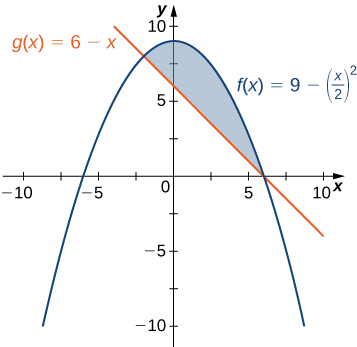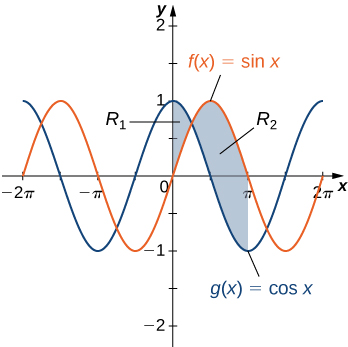# 6.1 Areas between curves  (Page 2/4)

 Page 2 / 4

If $R$ is the region bounded by the graphs of the functions $f\left(x\right)=\frac{x}{2}+5$ and $g\left(x\right)=x+\frac{1}{2}$ over the interval $\left[1,5\right],$ find the area of region $R.$

$12$ units 2

In [link] , we defined the interval of interest as part of the problem statement. Quite often, though, we want to define our interval of interest based on where the graphs of the two functions intersect. This is illustrated in the following example.

## Finding the area of a region between two curves 2

If $R$ is the region bounded above by the graph of the function $f\left(x\right)=9-{\left(x\text{/}2\right)}^{2}$ and below by the graph of the function $g\left(x\right)=6-x,$ find the area of region $R.$

The region is depicted in the following figure.This graph shows the region below the graph of f ( x ) and above the graph of g ( x ) .

We first need to compute where the graphs of the functions intersect. Setting $f\left(x\right)=g\left(x\right),$ we get

$\begin{array}{ccc}\hfill f\left(x\right)& =\hfill & g\left(x\right)\hfill \\ \\ \hfill 9-{\left(\frac{x}{2}\right)}^{2}& =\hfill & 6-x\hfill \\ \hfill 9-\frac{{x}^{2}}{4}& =\hfill & 6-x\hfill \\ \hfill 36-{x}^{2}& =\hfill & 24-4x\hfill \\ \hfill {x}^{2}-4x-12& =\hfill & 0\hfill \\ \hfill \left(x-6\right)\left(x+2\right)& =\hfill & 0.\hfill \end{array}$

The graphs of the functions intersect when $x=6$ or $x=-2,$ so we want to integrate from $-2$ to $6.$ Since $f\left(x\right)\ge g\left(x\right)$ for $-2\le x\le 6,$ we obtain

$\begin{array}{cc}\hfill A& ={\int }_{a}^{b}\left[f\left(x\right)-g\left(x\right)\right]dx\hfill \\ & ={\int }_{-2}^{6}\left[9-{\left(\frac{x}{2}\right)}^{2}-\left(6-x\right)\right]dx={\int }_{-2}^{6}\left[3-\frac{{x}^{2}}{4}+x\right]dx\hfill \\ & ={\left[3x-\frac{{x}^{3}}{12}+\frac{{x}^{2}}{2}\right]\phantom{\rule{0.2em}{0ex}}|}_{-2}^{6}=\frac{64}{3}.\hfill \end{array}$

The area of the region is $64\text{/}3$ units 2 .

If R is the region bounded above by the graph of the function $f\left(x\right)=x$ and below by the graph of the function $g\left(x\right)={x}^{4},$ find the area of region $R.$

$\frac{3}{10}$ unit 2

## Areas of compound regions

So far, we have required $f\left(x\right)\ge g\left(x\right)$ over the entire interval of interest, but what if we want to look at regions bounded by the graphs of functions that cross one another? In that case, we modify the process we just developed by using the absolute value function.

## Finding the area of a region between curves that cross

Let $f\left(x\right)$ and $g\left(x\right)$ be continuous functions over an interval $\left[a,b\right].$ Let $R$ denote the region between the graphs of $f\left(x\right)$ and $g\left(x\right),$ and be bounded on the left and right by the lines $x=a$ and $x=b,$ respectively. Then, the area of $R$ is given by

$A={\int }_{a}^{b}|f\left(x\right)-g\left(x\right)|dx.$

In practice, applying this theorem requires us to break up the interval $\left[a,b\right]$ and evaluate several integrals, depending on which of the function values is greater over a given part of the interval. We study this process in the following example.

## Finding the area of a region bounded by functions that cross

If R is the region between the graphs of the functions $f\left(x\right)=\text{sin}\phantom{\rule{0.2em}{0ex}}x$ and $g\left(x\right)=\text{cos}\phantom{\rule{0.2em}{0ex}}x$ over the interval $\left[0,\pi \right],$ find the area of region $R.$

The region is depicted in the following figure.The region between two curves can be broken into two sub-regions.

The graphs of the functions intersect at $x=\pi \text{/}4.$ For $x\in \left[0,\pi \text{/}4\right],$ $\text{cos}\phantom{\rule{0.2em}{0ex}}x\ge \text{sin}\phantom{\rule{0.2em}{0ex}}x,$ so

$|f\left(x\right)-g\left(x\right)|=|\text{sin}\phantom{\rule{0.2em}{0ex}}x-\text{cos}\phantom{\rule{0.2em}{0ex}}x|=\text{cos}\phantom{\rule{0.2em}{0ex}}x-\text{sin}\phantom{\rule{0.2em}{0ex}}x.$

On the other hand, for $x\in \left[\pi \text{/}4,\pi \right],$ $\text{sin}\phantom{\rule{0.2em}{0ex}}x\ge \text{cos}\phantom{\rule{0.2em}{0ex}}x,$ so

$|f\left(x\right)-g\left(x\right)|=|\text{sin}\phantom{\rule{0.2em}{0ex}}x-\text{cos}\phantom{\rule{0.2em}{0ex}}x|=\text{sin}\phantom{\rule{0.2em}{0ex}}x-\text{cos}\phantom{\rule{0.2em}{0ex}}x.$

Then

$\begin{array}{cc}\hfill A& ={\int }_{a}^{b}|f\left(x\right)-g\left(x\right)|dx\hfill \\ & ={\int }_{0}^{\pi }|\text{sin}\phantom{\rule{0.2em}{0ex}}x-\text{cos}\phantom{\rule{0.2em}{0ex}}x|dx={\int }_{0}^{\pi \text{/}4}\left(\text{cos}\phantom{\rule{0.2em}{0ex}}x-\text{sin}\phantom{\rule{0.2em}{0ex}}x\right)dx+{\int }_{\pi \text{/}4}^{\pi }\left(\text{sin}\phantom{\rule{0.2em}{0ex}}x-\text{cos}\phantom{\rule{0.2em}{0ex}}x\right)dx\hfill \\ & ={\left[\text{sin}\phantom{\rule{0.2em}{0ex}}x+\text{cos}\phantom{\rule{0.2em}{0ex}}x\right]\phantom{\rule{0.2em}{0ex}}|}_{0}^{\pi \text{/}4}+{\left[\text{−}\text{cos}\phantom{\rule{0.2em}{0ex}}x-\text{sin}\phantom{\rule{0.2em}{0ex}}x\right]\phantom{\rule{0.2em}{0ex}}|}_{\pi \text{/}4}^{\pi }\hfill \\ & =\left(\sqrt{2}-1\right)+\left(1+\sqrt{2}\right)=2\sqrt{2}.\hfill \end{array}$

The area of the region is $2\sqrt{2}$ units 2 .

If R is the region between the graphs of the functions $f\left(x\right)=\text{sin}\phantom{\rule{0.2em}{0ex}}x$ and $g\left(x\right)=\text{cos}\phantom{\rule{0.2em}{0ex}}x$ over the interval $\left[\pi \text{/}2,2\pi \right],$ find the area of region $R.$

$2+2\sqrt{2}$ units 2

## Finding the area of a complex region

Consider the region depicted in [link] . Find the area of $R.$Two integrals are required to calculate the area of this region.

As with [link] , we need to divide the interval into two pieces. The graphs of the functions intersect at $x=1$ (set $f\left(x\right)=g\left(x\right)$ and solve for x ), so we evaluate two separate integrals: one over the interval $\left[0,1\right]$ and one over the interval $\left[1,2\right].$

Over the interval $\left[0,1\right],$ the region is bounded above by $f\left(x\right)={x}^{2}$ and below by the x -axis, so we have

${A}_{1}={\int }_{0}^{1}{x}^{2}dx={\frac{{x}^{3}}{3}\phantom{\rule{0.2em}{0ex}}|}_{0}^{1}=\frac{1}{3}.$

Over the interval $\left[1,2\right],$ the region is bounded above by $g\left(x\right)=2-x$ and below by the $x\text{-axis,}$ so we have

${A}_{2}={\int }_{1}^{2}\left(2-x\right)dx={\left[2x-\frac{{x}^{2}}{2}\right]\phantom{\rule{0.2em}{0ex}}|}_{1}^{2}=\frac{1}{2}.$

Adding these areas together, we obtain

$A={A}_{1}+{A}_{2}=\frac{1}{3}+\frac{1}{2}=\frac{5}{6}.$

The area of the region is $5\text{/}6$ units 2 .

f(x) = x-2 g(x) = 3x + 5 fog(x)? f(x)/g(x)
fog(x)= f(g(x)) = x-2 = 3x+5-2 = 3x+3 f(x)/g(x)= x-2/3x+5
diron
pweding paturo nsa calculus?
jimmy
how to use fundamental theorem to solve exponential
find the bounded area of the parabola y^2=4x and y=16x
what is absolute value means?
Chicken nuggets
Hugh
🐔
MM
🐔🦃 nuggets
MM
(mathematics) For a complex number a+bi, the principal square root of the sum of the squares of its real and imaginary parts, √a2+b2 . Denoted by | |. The absolute value |x| of a real number x is √x2 , which is equal to x if x is non-negative, and −x if x is negative.
Ismael
find integration of loge x
find the volume of a solid about the y-axis, x=0, x=1, y=0, y=7+x^3
how does this work
Can calculus give the answers as same as other methods give in basic classes while solving the numericals?
log tan (x/4+x/2)
Rohan
Rohan
y=(x^2 + 3x).(eipix)
Claudia
Ismael
A Function F(X)=Sinx+cosx is odd or even?
neither
David
Neither
Lovuyiso
f(x)=1/1+x^2 |=[-3,1]
apa itu?
fauzi
determine the area of the region enclosed by x²+y=1,2x-y+4=0
Hi
MP
Hi too
Vic
hello please anyone with calculus PDF should share
Which kind of pdf do you want bro?
Aftab
hi
Abdul
can I get calculus in pdf
Abdul
explain for me
Usman
How to use it to slove fraction
Hello please can someone tell me the meaning of this group all about, yes I know is calculus group but yet nothing is showing up
Shodipo
You have downloaded the aplication Calculus Volume 1, tackling about lessons for (mostly) college freshmen, Calculus 1: Differential, and this group I think aims to let concerns and questions from students who want to clarify something about the subject. Well, this is what I guess so.
Jean
Im not in college but this will still help
nothing
how en where can u apply it
Migos
how can we scatch a parabola graph
Ok
Endalkachew
how can I solve differentiation?
with the help of different formulas and Rules. we use formulas according to given condition or according to questions
CALCULUS
For example any questions...
CALCULUS
v=(x,y) وu=(x,y ) ∂u/∂x* ∂x/∂u +∂v/∂x*∂x/∂v=1
log tan (x/4+x/2)
Rohan
what is the procedures in solving number 1?By Rachel WoolardBy OpenStaxBy OpenStaxBy OpenStaxBy Jazzycazz JacksonBy RhodesBy Richley CrapoBy Jazzycazz JacksonBy OpenStaxBy Eddie Unverzagt